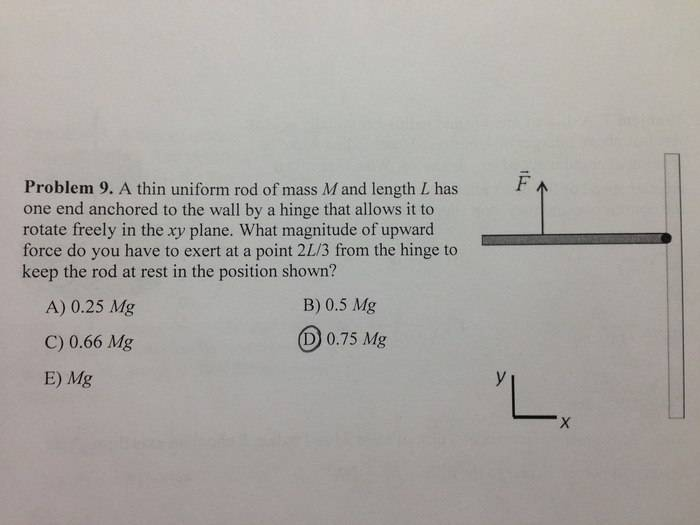# Hanging Rod - Torque Question

Hey, folks! So upon studying for my Physics final tomorrow, I came across this question on the practice exam, which I know the answer is D from the solution key. However, I'm not sure how to get that for the answer.

1. Homework Statement## Homework Equations

τ = rA × Fnet
LA = Ltrans, A + Lrot
Ltrans = rcm × Ptot
Lrot = r1 × p1 + r2 × p2 + ...

## The Attempt at a Solution

I personally don't entirely know where to start. I'm going to guess this is a torque-related question, but I'm not sure if that's the right way to go at it.

## Answers and Replies

PhanthomJay
Science Advisor
Homework Helper
Gold Member
The rod is at rest when the force F is applied. What can you say about what the sum of the torques about the hinge must be for bodies at rest? No need to get involved with momentum

The net torque would equal zero.

haruspex
Science Advisor
Homework Helper
Gold Member
2020 Award
The net torque would equal zero.
So what are the torques? What equation do you get for a zero sum?

Would it just be gravity and the force pushing the rod upwards?

I asked one of the graduate teaching assistants about this and he was able to help me figure this out. I simply forgot that the center of mass is where gravity is pulling down on the rod.